# Hellinger distance

(diff) ← Older revision | Latest revision (diff) | Newer revision → (diff)

A distance between probability measures, expressed in terms of the Hellinger integral. Suppose that on a measurable space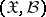a family of probability measures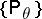,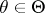, is given that are all absolutely continuous relative to some-finite measureon.

The Hellinger distance between two measuresand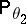(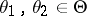) is defined by the formula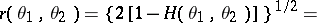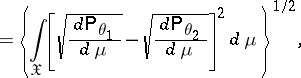where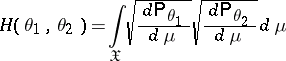is the Hellinger integral. The Hellinger distance does not depend on the choice of the measureand has the following properties:

1)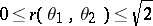;

2)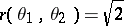if and only if the measures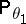andare mutually singular;

3)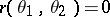if and only if.

Let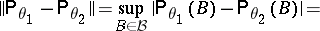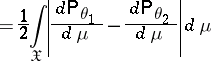be the distance in variation between the measuresand. Then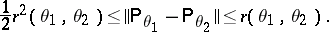How to Cite This Entry:
Hellinger distance. Encyclopedia of Mathematics. URL: http://encyclopediaofmath.org/index.php?title=Hellinger_distance&oldid=16453
This article was adapted from an original article by M.S. Nikulin (originator), which appeared in Encyclopedia of Mathematics - ISBN 1402006098. See original article